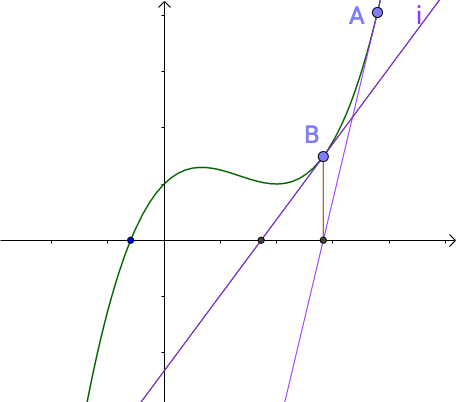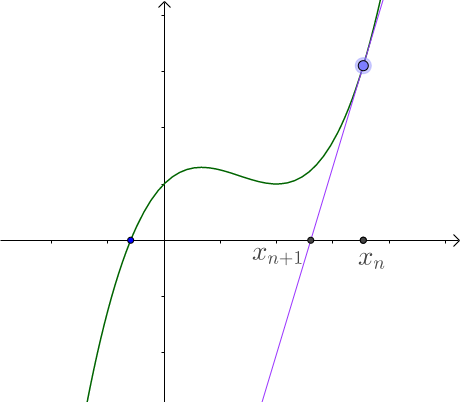# Sqrtx——python——牛顿迭代法详解

“””

Implement int sqrt(int x).
Compute and return the square root of x.
“””B点比之前A点更接近曲线的根点。那么经过多次迭代后会越来越接近曲线的根。${x}_{n}$$x_{n}$点的切线方程为：$f\left({x}_{n}\right)+{f}^{{}^{\prime }}\left({x}_{n}\right)\left(x-{x}_{n}\right)$$f(x_{n})+f^{'}(x_{n})(x-x_{n})$

$x=\frac{\left({x}_{n}+\frac{a}{{x}_{n}}\right)}{2}$

class Solution(object):
def mySqrt(self, x):
"""
:type x: int
:rtype: int
"""
result = 1.0
while abs(result * result - x) > 0.1:
result = (result + x / result) / 2
return int(result)

$|{x}_{n}-\frac{\left({x}_{n}+\frac{a}{{x}_{n}}\right)}{2}|-|{x}_{n+1}-\frac{\left({x}_{n}+\frac{a}{{x}_{n}}\right)}{2}|>0$

${x}_{n+1}={x}_{n}-\frac{f\left({x}_{n}\right)}{{f}^{{}^{\prime }}\left({x}_{n}\right)}$

ps：这题虽然简单，但是确实让我好学了一把牛顿迭代法！©️2019 CSDN 皮肤主题: 大白 设计师: CSDN官方博客